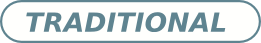# $LT^2C^2$ : A language of thought with Turing-computable Kolmogorov complexity

• Sergio Romano Department of Computer Science, FCEN, University of Buenos Aires
• Mariano Sigman Laboratory of Integrative Neuroscience, Physics Department, FCEN, University of Buenos Aires.
• Santiago Figueira Laboratory of Integrative Neuroscience, Physics Department, FCEN, University of Buenos Aires
Keywords: Complexity, Psychology

### Abstract

In this paper, we present a theoretical effort to connect the theory of program size to psychology by implementing a concrete language of thought with Turing-computable Kolmogorov complexity ($LT^2C^2$ ) satisfying the following requirements: 1) to be simple enough so that the complexity of any given finite binary sequence can be computed, 2) to be based on tangible operations of human reasoning (printing, repeating,. . . ), 3) to be sufficiently powerful to generate all possible sequences but not too powerful as to identify regularities which would be invisible to humans. We first formalize $LT^2C^2$ , giving its syntax and semantics, and defining an adequate notion of program size. Our setting leads to a Kolmogorov complexity function relative to $LT^2C^2$ which is computable in polynomial time, and it also induces a prediction algorithm in the spirit of Solomonoff’s inductive inference theory. We then prove the efficacy of this language by investigating regularities in strings produced by participants attempting to generate random strings. Participants had a profound understanding of randomness and hence avoided typical misconceptions such as exaggerating the number of alternations. We reasoned that remaining regularities would express the algorithmic nature of human thoughts, revealed in the form of specific patterns. Kolmogorov complexity relative to $LT^2C^2$ passed three expected tests examined here: 1) human sequences were less complex than control PRNG sequences, 2) human sequences were not stationary showing decreasing values of complexity resulting from fatigue 3) each individual showed traces of algorithmic stability since fitting of partial data was more effective to predict subsequent data than average fits. This work extends on previous efforts to combine notions of Kolmogorov complexity theory and algorithmic information theory to psychology, by explicitly proposing a language which may describe the patterns of human thoughts.

Received: 12 December 2012,  Accepted: 3 February 2013; Edited by: G. Mindlin; DOI: http://dx.doi.org/10.4279/PIP.050001

Cite as: S Romano, M Sigman, S Figueira, Papers in Physics 5, 050001 (2013)Published
2013-03-01
How to Cite
Romano, S., Sigman, M., & Figueira, S. (2013). $LT^2C^2$ : A language of thought with Turing-computable Kolmogorov complexity. Papers in Physics, 5, 050001. https://doi.org/10.4279/pip.050001
Issue
Section
Articles